# FAQ Number 2

## What Are the Effects of the Interest Rate, Volatility, and Other Parameters in Both Option Value and the Decision Rule?

Answer with “Timing Suite”, that originated the charts below, exploiting the analogy of real options problem with American call option.
For each parameter (ex.: volatility) are showed both charts, the option value and the threshold. The charts are animated in order to show the variation with the parameters.
Threshold is the earlier exercise decision rule: invest immediately if the underlying asset (project value V) is at or above the threshold line. The threshold is the strategy of earlier exercise that maximize the option value.

What is the effect of an increasing uncertainty on real options? Let us vary the parameter of the underlying asset uncertainty, the volatility s.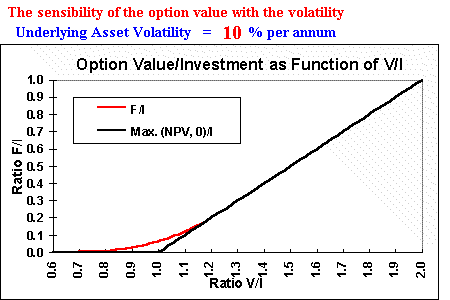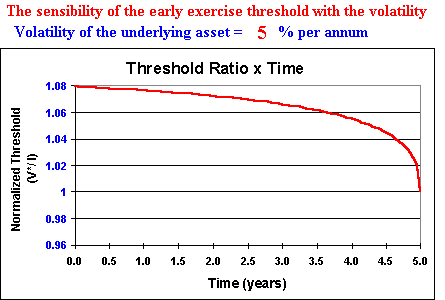So, the volatility increases the option value and the threshold. The wait and see strategy has more value with the uncertainty.

What is the effect of the interest rate in both the option value and the threshold for the immediate investment? See the charts below.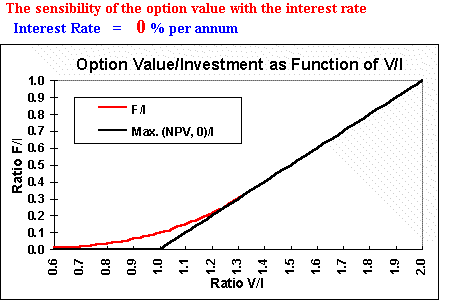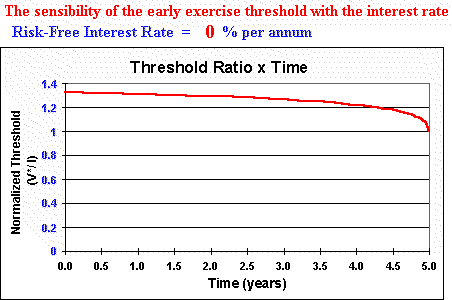The interest rate also increases both option value and threshold. Think that you leave in the bank the money to pay an eventual option exercise. If the interest rate increases, you have less incentive to exercise the option using that money.
Or in terms of present value, exercising option late instead earlier is more attractive because the present value of the exercise price (the investment cost) is lower for the delay strategy.

The picture below show the effect of the time to expiration in the option value.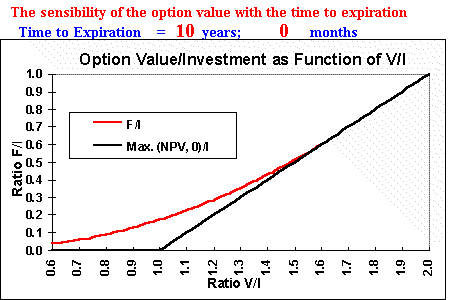As expected, higher time to expiration means higher option value. But is very known that there is an upper bound for this case. The perpetual American option (with infinite time to expiration, as an option to develop a land) has a known analytic solution that is the upper bound for the option with the expiration time. See Dixit & Pindyck (1994, chapter 5).

The pictures below show the effect of the dividend yield (or convenience yield for commodities) d on both the option value and the decision rule (thresholds).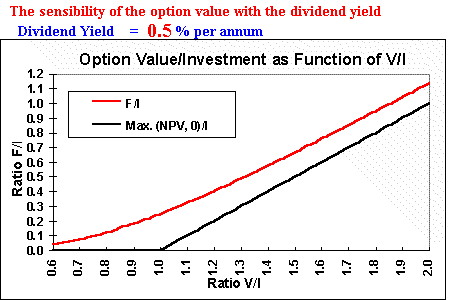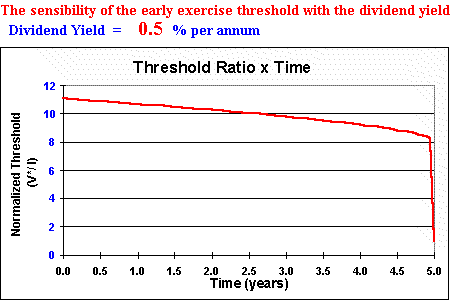The dividend (convenience) yield d has an opposite effect compared with the interest rate. Dividend yield is like an opportunity cost of not holding the underlying asset. So, only who own the underlying asset earn the flow of benefits associated (dividend yield). Higher d means lower value to waiting to invest, so the threshold is lower and the live option has lower value.

"Timing Suite" spreadsheets show these and other sensibilities. It comprises three spreadsheets that uses a simple model analogy of real options problem with American call option, including the case for two source of uncertainty and the option to switch use or to abandon. See the real options software webpage.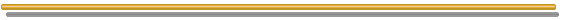Go to the Next FAQ: 3) Where the real options value comes from? Why real options value is different of the static net present value (NPV)?

Back to the FAQ's ListBack to Contents